# Cotangent: Definition & Formula

Lesson Transcript
Instructor: Ellen Manchester
The cotangent is the reciprocal function, or 'flipped' version, of a given tangent. Learn the formula for cotangents and understand their application in trigonometry and unit circles. Updated: 10/17/2021

## Trigonometry Importance

The application of trigonometric (trig) functions is widely used in our world. These functions are one of the basic math functions in areas like triangulation, which is used in criminal investigations and cell service. They're also used in navigation, surveying, computer graphics, and music theory. Did you know the shape of a vibrating guitar string is the same shape as the sine wave? This isn't a coincidence. In this lesson, we will be learning about the trigonometric function cotangent.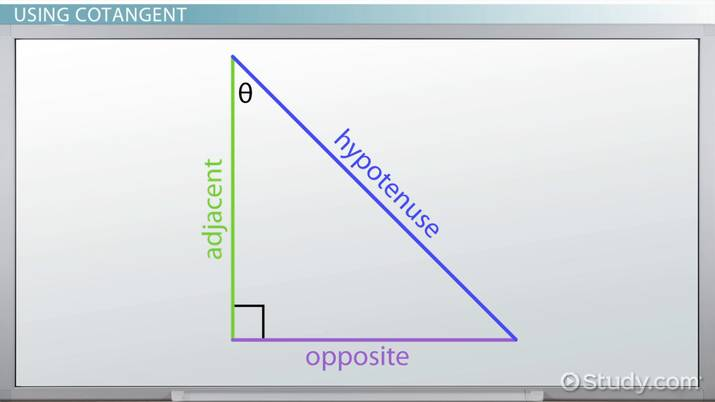An error occurred trying to load this video.

Try refreshing the page, or contact customer support.

Coming up next: Cubic Function: Definition, Formula & Examples

### You're on a roll. Keep up the good work!

Replay
Your next lesson will play in 10 seconds
• 0:04 Trigonometry Importance
• 0:33 Using Cotangent
• 1:23 Applications of Cotangent
• 2:57 Unit Circle Used to…
• 5:12 Lesson Summary
Save Save

Want to watch this again later?

Timeline
Autoplay
Autoplay
Speed Speed

## Using Cotangent

To learn about cotangent, we must first review what a tangent is. The tangent function can be found by comparing the opposite side over the adjacent side. This is also viewed by comparing the sine over the cosine functions. Using this triangle that you can see below, we can determine the lengths of the sides by comparing the sides based on the angle we're using.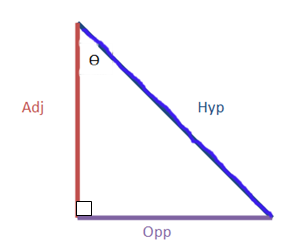Tangent is sine/cosine, or opposite/adjacent. We can see why by calculating using sine/cosine. You can see how these are calculated below.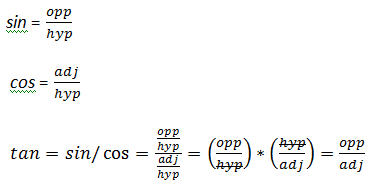This set up is important because cotangent is the reciprocal function of tangent. Cotangent is just the 'flipped' version of tangent, as you can see with the equation below.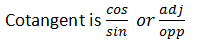## Applications of Cotangent

Cotangent is used the same way the sine, cosine, and tangent functions are used. You can use them based on a right triangle, using the opposite and adjacent sides of the triangle, or you can use it based on the unit circle, which shows the angles in radians.

If we set up a 45-45-90 triangle, we can find the cotangent easily, as you can see below: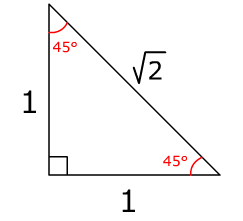Notice the 45-degree angles have sides opposite of 1 and the hypotenuse is square root of 2. To find the cotangent of the 45-degree angle, it is adjacent/opposite, or 1/1, or 1.

Using the 30-60-90 triangle, the cotangent of the 30-degree angle is sqrt(3)/1, or square root of 3. The cotangent of the 60-degree angle is 1/sqrt(3).

If I wanted to find the height of a particular tree based on the shadow it throws when the sun is at a 30-degree angle, we can find this using cotangent. We'll use the 30-60-90 triangle to find this.

The cotangent of a 30-degree angle is square root of 3. Setting up our cotangent ratio, we can calculate the height of the tree this way: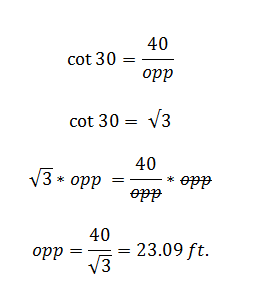If a contractor is standing 20 feet from a wall and is looking at a 45-degree angle to the top of the wall, he can find the height of the wall.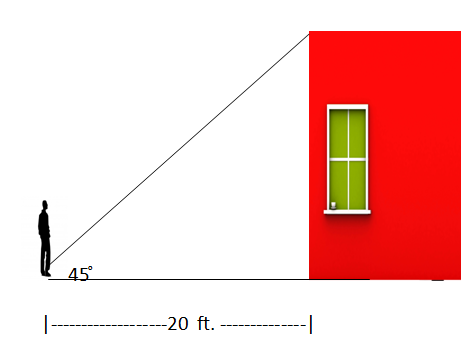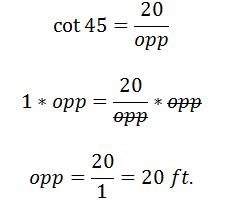To unlock this lesson you must be a Study.com Member.

### Register to view this lesson

Are you a student or a teacher?